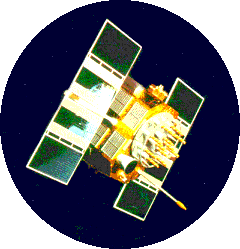The difficult takes time, the impossible can happen overnight. Author Unknown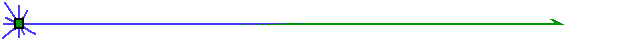# Satellites Everywhere...

### "Watching over You"Homework Quiz

Objectives:

• Be able to derive orbital velocity from Newton's second law and the law of gravitation.
• Be able to determine the energy and angular momentum requirements to transfer from one circular orbit to another.
• Be able to calculate the escape velocity of an object from the surface of a planet and from satellite orbits.
• Be able to derive escape velocity from the total energy equation.
• Understand the difference between escape velocity and orbital velocity.
• Understand what is meant by the Satellite Orbit Paradox.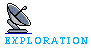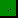Nothing YetNothing Yet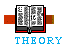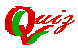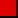Orbital SpeedsOrbital Transfers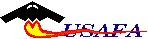Click here to return to the Mission Planning Page